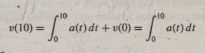Velocity from an Accelerometer

An accelerometer measures acceleration and is used in aircraft, rockets, and other vehicles to estimate the vehicle’s velocity and displacement. The accelerometer integrates the  acceleration signal to produce an estimate of the velocity, and it integrates the velocity estimate to produce an estimate of displacement. Suppose the vehicle starts from rest at time t = 0 and that its measured acceleration is giverr in the following table.
a) Estimate the velocity after 10 s.
) Estimate the velocity at the times t = I, 2, … , 10 s.
c) Check your program by using a case that can be solved analytically.• Solution
a) We must use the trapz function here, because the acceleration is given as a table of
values. We cannot use the quad or quadl functions. The relation between velocity and
acceleration isThe script file follows.
: = [0:10];
a = [0;2,4,7,11,17,24,32,41,48,51];
vf = trapz(t,a)
The answer for the final velocity is given by vf and-is 211.5 mls .
. (b) To find the velocity at the times t = 1,2, … , 10,we can use the fact that v(tJ) = 0
and write (8.1-2) asfor k = 2, 3, … , II. (Note that there are II values in the sequence t = 0, 1, 2, … , 10.
Thus tll corresponds to 1. = 10.)
(8.2-1)
t = [0:10];
a = [0,2,4,7,11,17,24,32,41,48,51];
v(l) = 0;
for k = [2:11]
v(k) = trapz(t(l:k),a(l:k));
end
disp([t’,v’])
The preceding method uses more calculations than necessary because it does not take
advantage of the velocity value calculated ID the previous pass through the for loop. The

following script file is thus more efficient. It is based on (8.1-2), which can be written
follows by substituting te for 0 and tHI for b.t = [0:10];
a = 10,2,4,7,11,17,24,32,41,48,51];
v(l) = 0;
for k = [1:10]
v(k+1) = trapz(t(k:k+1),a(k:k+1))+v(k);
end
di sp ( [t’ ,v’ ] )

For either method the answers are those given in the following table:(c) Because the trapezoidal rule uses straight-line segments to connect the data points,
it will give the exact solution for any panel width when the integrand is a linear function.
So we can use the linear acceleration function aCt) = t to test the program. The velocity
is given byThe velocity at t = lO is SO. The following script file can be used to check the method
used In-part (b):
t·= [0:10];
a = t;
v(l) = 0;
for k = [1:10]
v(k+1) = trapz(t(k:k+1),a(k:k+1))+v(k);
end
disp(v(ll) )
When this file is run it produces the answer v (11) = 50, which is correct.